# Dense Pythagorean Triples -- A Cute Problem in Arithmetic Geometry

### 16 Nov 2021 - Tags: pretty-pictures , sage

I follow a lot of other math blogs, and it’s always fun reading about what various people are thinking about. Particularly fun for me is when blogs pose problems to their readers, and the other day Shiva Kintali posted a really cute problem: For any rational numbers $\alpha \lt \beta \in (0,1)$, can we always find a pythagorean triple $a^2 + b^2 = c^2$ so that $\alpha \lt \frac{a}{b} \lt \beta$?

As soon as I read this problem I was reminded of a really clever trick that is one of the simplest examples in arithmetic geometry. I probably saw this earlier, but I really internalized the idea after seeing it first in a lecture of Richard E. Borcherds (here) then again in the opening chapter of Stillwell’s Mathematics and its History. I’ll summarize the idea here, but for more information you should check out either of these (excellent!) references.

Rewrite $a^2 + b^2 = c^2$ as $\left ( \frac{a}{c} \right )^2 + \left ( \frac{b}{c} \right )^2 = 1$. From this, it’s clear that pythagorean triples are in bijection with rational points on the unit circle. Of course, there are some “obvious” rational points on the circle, and here I believe it’s traditional to choose $(-1,0)$, but any rational point will do. Now it’s time for the geometric insight: Pick a rational point on the circle. Then the line from $(-1,0)$ to that point will have rational slope. Conversely, any line through $(-1,0)$ with rational slope will intersect the unit circle in some rational point1.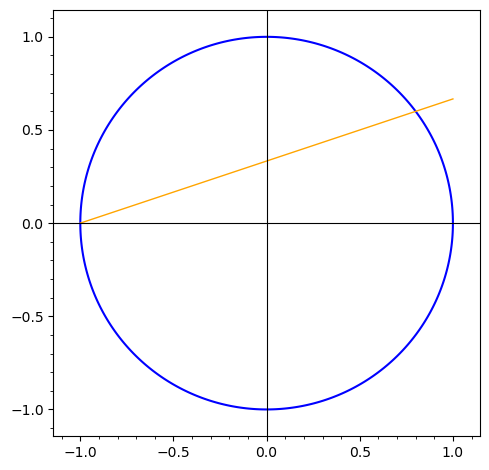This means we can compeltely characterize pythagorean triples by rational slopes. In the language of algebraic geometry, we say that the affine line is birationally equivalent to the circle.

Now, with this idea in mind, can you answer Kintali’s question? That is, can you show that for any $\alpha \lt \beta \in (0,1)$ there is some $a^2 + b^2 = c^2$ with $\alpha \lt \frac{a}{b} \lt \beta$?

I’m going to talk about the solution now, but this is a particularly satisfying problem to solve for yourself, and I recommend you give it a try!

Ok, so what’s the plan? Well we’re intersted in $\frac{a}{b}$ so let’s take our desired equation $a^2 + b^2 = c^2$ and turn it into $\left ( \frac{a}{b} \right )^2 + 1 = \left ( \frac{c}{b} \right )^2$. If we write $x = \frac{a}{b}$ and $y = \frac{c}{b}$, then we’re looking for rational points on the hyperbola $y^2 - x^2 = 1$: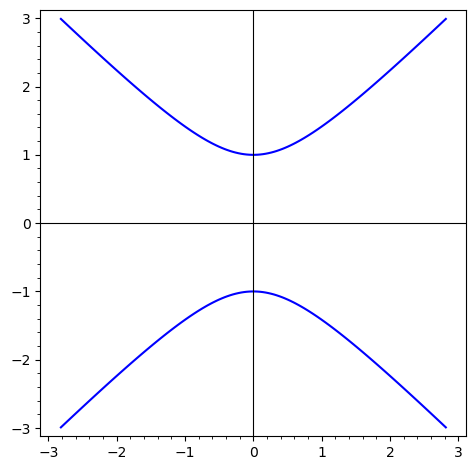Following the earlier example, there’s an “obvious” rational point on this curve: $(0,1)$, and from here we can get every rational point on the curve by looking at the lines of rational slope $t$ through this point2: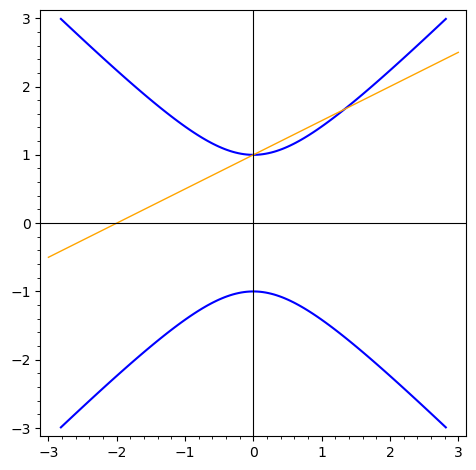But now the answer to the question is entirely obvious! Let’s fix some $\alpha \lt \beta \in (0,1)$. Then we want to know if there’s a rational point on this curve whose $x$ coordinate lies in that range (remember, we set $x = \frac{a}{b}$):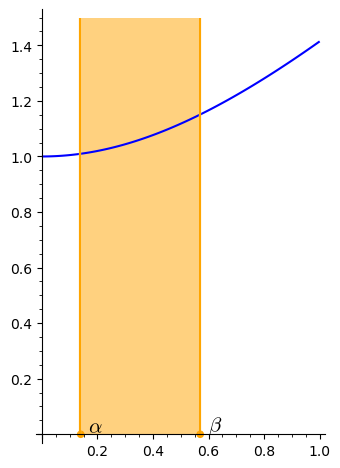Of course, from this picture we would expect there to be a plethora of rational points on the curve whose $x$ coordinate lies in the shaded region. All we need to do is choose a slope $t$ so that the line through $(0,1)$ of slope $t$ intersects the blue cuve somewhere in the shaded region!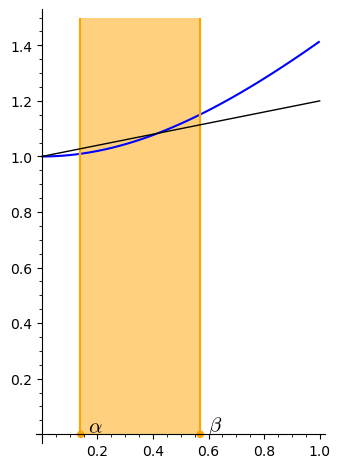It’s at this point that we can (conceptually) stop. We know the answer to Kintali’s question is “yes”. But it’s fun to work out the details so that we can really believe the answer. After all, this machinery is really making some kind of prediction, and should try to test our theory in some special cases!

Again, I encourage you to try and work out the computational details yourself! Now is the time to give it a go ^_^

Ok, so we’re interested in where lines of (rational) slope $t$ through $(0,1)$ intersect the curve $y^2 - x^2 = 1$. That is, we’re interested in the solutions $(x,y)$ to the system of equations

$y = tx + 1$ $y^2 - x^2 = 1$

for various choices of $t \in \mathbb{Q}$.

Of course, we can substitute the first equation into the second, and solve for $x$ to see

$x = \frac{-2t}{t^2 -1 }.$

From here, we can get $y$ by using $x$ and the first equation, and we see that the rational points on our curve are exactly

$\left ( \frac{a}{b}, \frac{c}{b} \right ) = (x,y) = \left ( \frac{-2t}{t^2 - 1}, \frac{-t^2-1}{t^2-1} \right )$

for any $t \in \mathbb{Q}$ (except $\pm 1$).

So now to solve our problem, what do we need to do? Well, given $\alpha \lt \beta$, we want to find some $t \in \mathbb{Q}$ so that

$\frac{a}{b} = x(t) = \frac{-2t}{t^2 - 1} \in (\alpha, \beta)$

Then, if all is right with the world, we’ll have successfully found a pythagorean triple $a^2 + b^2 = c^2$ with $\alpha \lt \frac{a}{b} \lt \beta$. How far we’ve come!

Now, how do we choose such a $t$? Well, if we plot $x(t)$, we see as $t$ goes from $-1$ to $1$, $x$ increases monotonically from $-\infty$ to $\infty$, so all we need to do is invert this function and we’ll win!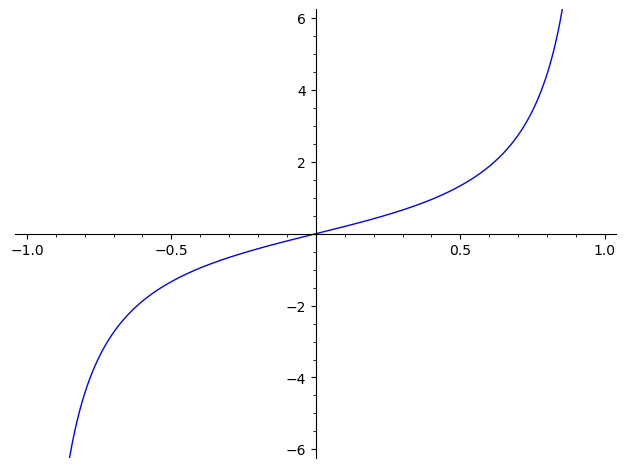But this is easy to do, and we see that

$t(x) = \frac{-1 + \sqrt{1 + x^2}}{x}$

gets the job done.

In particular, for some $\alpha \lt \beta$, we can choose any $t \in \mathbb{Q}$ with

$\frac{-1 + \sqrt{1 + \alpha^2}}{\alpha} \lt t \lt \frac{-1 + \sqrt{1 + \beta^2}}{\beta}$

then

$\left ( \frac{a}{b}, \frac{c}{b} \right ) = (x,y) = \left ( \frac{-2t}{t^2 - 1}, \frac{-t^2-1}{t^2-1} \right )$

will give us the desired triple $a^2 + b^2 = c^2$ with $\alpha \lt \frac{a}{b} \lt \beta$!

Now what would be really neat would be if somebody hacked together an interactive demo for this… Woops! My mouse slipped!

1. As a (fun?) exercise, you should prove both of these claims. One direction should be quite easy, and the other is only a little bit tricky.

As a hint, you might ask yourself: is it possible for a quandratic equation to have one rational root and one irrational root?

2. You might be wondering what happens when you choose a slope of $\pm 1$. If you’ve heard of projective geometry, where we add some “points at infinty” to make the math “cleaner”, this is one instance of that! It turns out that while the slopes $\pm 1$ don’t correspond to any points in the affine case, once we projectivize they correspond to “points at infinity” where the right of the top curve meets the left of the bottom curve (this is the point associated to a slope of $+1$) or where the left of the top curve meets the right of hte bottom curve (which is associated to the slope $-1$).